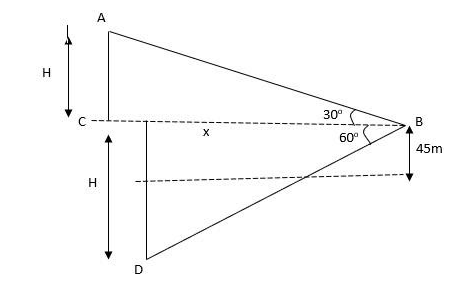QuestionAnswers

# The angle of elevation of a covering helicopter as see from a point $45m$ above a lake is ${{30}^{\circ }}$and the angle of depression of its reflection in the lake, as seen from the same point at the same time is ${{60}^{\circ }}$. Find the distance of the helicopter from the surface of the lake.

Hint:
Read the question and visualise what is given in the question, you will be able to draw a diagram then once you have drawn the figure, try to equate the value of distance of the helicopter from the surface of the lake from trigonometric knowledge that you have. Remember that the reflection of the helicopter will be in a straight line from the helicopter itself.

Complete step by step solution:
Step 1:
We begin the question by drawing the diagram as described in the question.Step 2:
We know, $\tan {{30}^{\circ }}$ $=$ $\dfrac{1}{\sqrt{3}}$.
In $\Delta ABC$,$\tan {{30}^{\circ }}$$=$ $\dfrac{AC}{BC}$$=$ $\dfrac{H-45}{x}$$=$$\dfrac{1}{\sqrt{3}}$ $......(i)$
Again, $\tan {{60}^{\circ }}$ $=$ $\sqrt{3}$
In $\Delta BCD$, $\tan {{60}^{0}}=\dfrac{CD}{BC}$$=$ $\dfrac{H+45}{x}$$=$$\sqrt{3}$$.....(ii)$
Step 3:
Dividing equation (i) and equation (ii),
$\dfrac{H-45}{H+45}$$=$ $\dfrac{1}{3}$
$\Rightarrow H=90 m$
Hence, the height h = distance of helicopter from the surface of the lake is$90m$.

Note:
Do not be confused as to why we have taken both the H as the same. We know that the reflection of an object in a plane/lake/river is formed at the same distance as the distance of the object from the mirror or lake. That is why H is the same. To solve such similar questions, remember the values of the trigonometric functions and that is how it becomes easier for you.